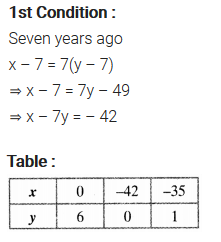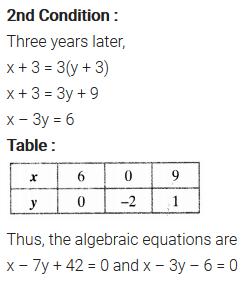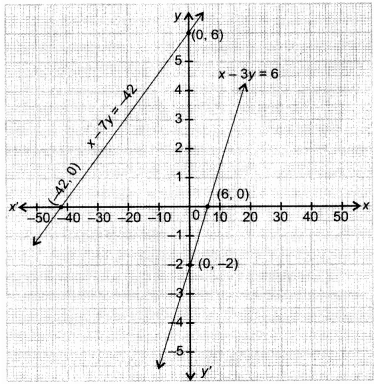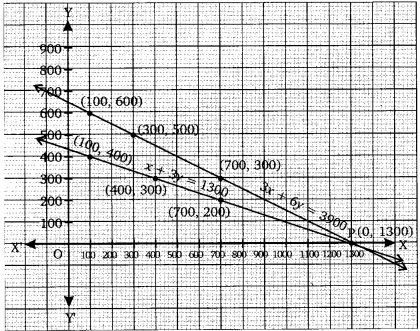• +91 9971497814
• info@interviewmaterial.com

# Pa.Linear Eq Ex-3.1 Interview Questions Answers

### Related Subjects

Question 1 :

Aftab tells his daughter, “Seven years ago, I was seven timesas old as you were then. Also, three years from now, I shall be three times asold as you will be”. Isn’t this interesting? Represent this situationalgebraically and graphically.

Solutions:

Let the present age of Aftab be ‘x’.

And, the present age of his daughter be ‘y’.Question 2 :

The coach of a cricket team buys 3 bats and 6balls for Rs.3900. Later, she buys another bat and 3 more balls of the samekind for Rs.1300. Represent this situation algebraically and geometrically.

Solutions:

Letus the cost of a bat be ‘Rs x’

And,the cost of a ball be ‘Rs y’

According to the question,

3x+6y = 3900

And x+3y = 1300

For, 3x+6y = 3900

Or x = (3900-6y)/3

The solution table is

 x 100 300 700 y 600 500 300

For, x+3y = 1300

Or x = 1300-3y

The solution table is

 x 100 400 700 y 400 300 200

The graphical representation is as follows.Question 3 :

The cost of 2 kg of apples and 1kg of grapeson a day was found to be Rs.160. After a month, the cost of 4 kg of apples and2 kg of grapes is Rs.300. Represent the situation algebraically andgeometrically.

Solutions:

Let the cost of 1 kg of apples be ‘Rs. x’

And, cost of 1 kg of grapes be ‘Rs. y’

According to the question,

2x+y = 160

And 4x+2y = 300

For, 2x+y = 160 or y = 160−2x, thesolution table is;

 x 80 60 40 y 0 40 80

For 4x+2y = 300 or y = (300-4x)/2, thesolution table is;

 x 20 60 40 y 110 30 70

The graphical representation is as follows;